Courses

# RD Sharma Solutions - Chapter 1 - Rational Numbers (Ex -1. 6), Class 8, Maths Class 8 Notes | EduRev

## RD Sharma Solutions for Class 8 Mathematics

Created by: Abhishek Kapoor

## Class 8 : RD Sharma Solutions - Chapter 1 - Rational Numbers (Ex -1. 6), Class 8, Maths Class 8 Notes | EduRev

The document RD Sharma Solutions - Chapter 1 - Rational Numbers (Ex -1. 6), Class 8, Maths Class 8 Notes | EduRev is a part of the Class 8 Course RD Sharma Solutions for Class 8 Mathematics.
All you need of Class 8 at this link: Class 8

Question 1: Verify the property: x × y = y × x by taking: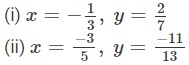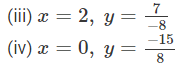We have to verify that x×y=y×x.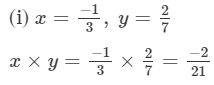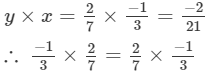Hence verified.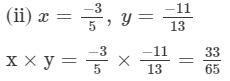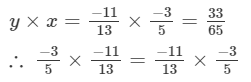Hence verified.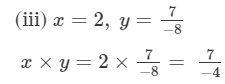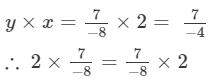Hence verified.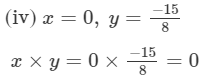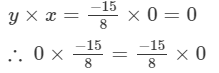Hence verified.

Question 2: Verify the property: x × (y × z) = (x × y) × z by taking: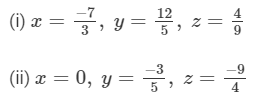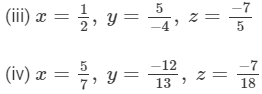We have to verify that x×(y×z)=(x×y)×z.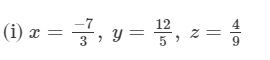x×(y×z)=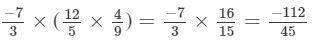(x×y)×z=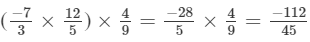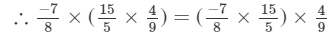Hence verified.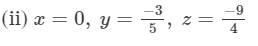x×(y×z)=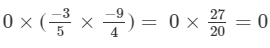(x×y)×z=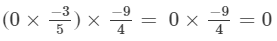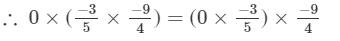Hence verified.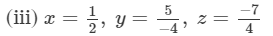x×(y×z)=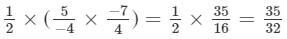(x×y)×z=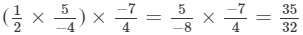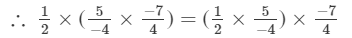Hence verified.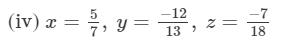x×(y×z)=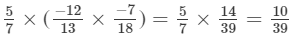(x×y)×z=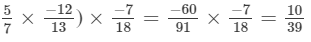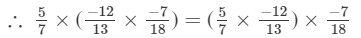Hence verified.

Question 3: Verify the property: x × (y + z) = x × y + x × z by taking: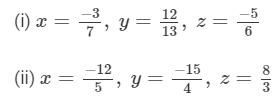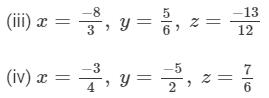We have to verify that  x×(y+z)=x×y+x×z.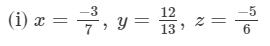x×(y+z)=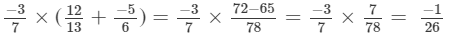x×y+x×z=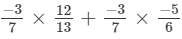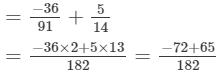= -1/26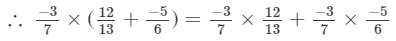Hence verified.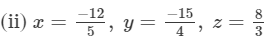x×(y+z)=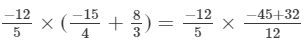=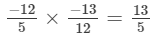x×y+x×z=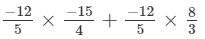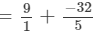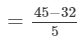= 13/5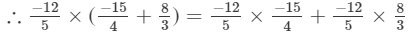Hence verified.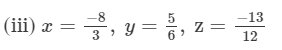x×(y+z)=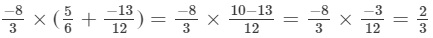x×y+x×z=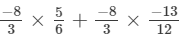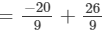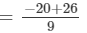= 2/3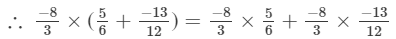Hence verified .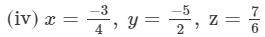x×(y+z)=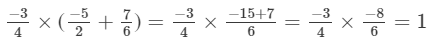x×y+x×z=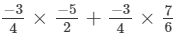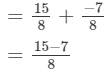= 1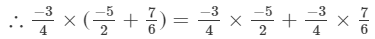Hence verified.

Question 4: Use the distributivity of multiplication of rational numbers over their addition to simplify: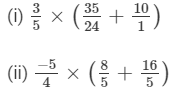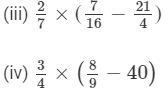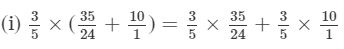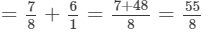(ii)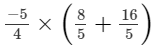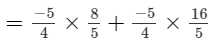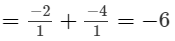(iii)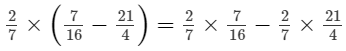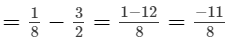(iv)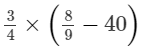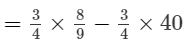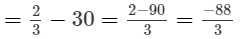Question 5: Find the multiplicative inverse (reciprocal) of each of the following rational numbers:
(i) 9

(ii) −7

(iii) 12/5

(iv) −7/9

(v) −3/−5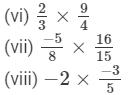(ix) −1
(x) 0/3
(xi) 1

(i) Multiplicative inverse (reciprocal) of 9 =1/9
(ii) Multiplicative inverse (reciprocal) of −7 = -1/7

(iii) Multiplicative inverse (reciprocal) of 12/5 = 5/12
(iv) Multiplicative inverse (reciprocal) of -7/9 = -9/7

(v) Multiplicative inverse (reciprocal) of -3/-5 = -5/-3 or 5/3

(vi) Multiplicative inverse (reciprocal) of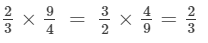(vii) Multiplicative inverse (reciprocal) of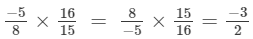(viii) Multiplicative inverse (reciprocal) of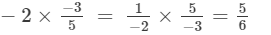(ix) Multiplicative inverse (reciprocal) of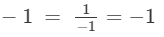(x) Multiplicative inverse (reciprocal) of 0/3 = 3/0 = Undefined
(ix) Multiplicative inverse (reciprocal) of 1 = 1/1 = 1

Question 6: Name the property of multiplication of rational numbers illustrated by the following statements: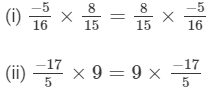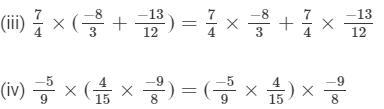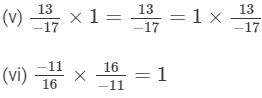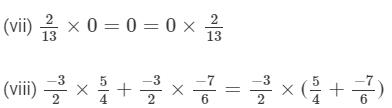(i) Commutative property
(ii) Commutative property
(iii) Distributivity of multiplication over addition
(iv) Associativity of multiplication
(v) Existence of identity for multiplication
(vi) Existence of multiplicative inverse
(vii) Multiplication by 0
(viii) Distributive property

Question 7: Fill in the blanks:
(i) The product of two positive rational numbers is always .....
(ii) The product of a positive rational number and a negative rational number is always .....
(iii) The product of two negative rational numbers is always .....
(iv) The reciprocal of a positive rational number is .....
(v) The reciprocal of a negative rational number is .....
(vi) Zero has ..... reciprocal.
(vii) The product of a rational number and its reciprocal is .....
(viii) The numbers ..... and ..... are their own reciprocals.
(ix) If a is reciprocal of b, then the reciprocal of b is .....
(x) The number 0 is ..... the reciprocal of any number.

(xi) Reciprocal of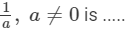(xii) (17 × 12)−1 = 17−1 × .....
(i) Positive
(ii) Negative
(iii) Positive
(iv) Positive
(v) Negative
(vi) No
(vii) 1
(viii) -1 and 1
(ix) a
(x) not
(xi) a
(xii) 12112-1

Question 8: Fill in the blanks: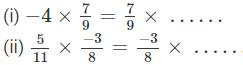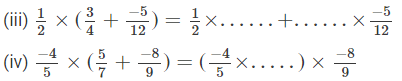(i) −4

x×y=y×x  (commutativity)

(ii) 5/11

x×y=y×x  (commutativity)

(iii) 3/4 ; 1/2

x×(y+z)=x×y+x×z  (distributivity of multiplication over addition)

(iv) 5/7

x×(y×z)=(x×y)×z  (associativity of multiplication )

91 docs

,

,

,

,

,

,

,

,

,

,

,

,

,

,

,

,

,

,

,

,

,

,

,

,

,

,

,

;